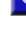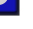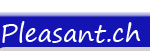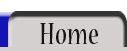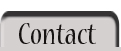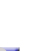And Eqv Imp Is Mod Not Or Xor =  (equal) <  (less-than) >  (greater-than) <=   (less-than or equal) >=  (greater-than or equal) <>   (not equal) +  (plus) &  (concatenate) -  (minus) /  (divide) \  (integer divide) *  (multiply) ^  (exponentiate)<